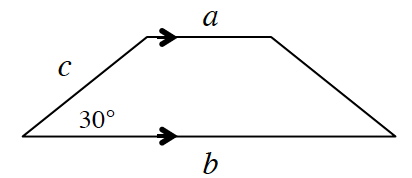### Home > CCG > Chapter Ch10 > Lesson 10.2.2 > Problem10-91

10-91.

Multiple Choice: Which expression represents the area of the trapezoid right?1. $\frac { c ( a + b ) } { 4 }$

1. $\frac { c ( a + b ) } { 2 }$

1. $\frac { b c } { 2 }$

1. $\frac { a + b + c } { 2 }$

1. None of these

(a)